Solutions   Share

What is Solutions

“A Clear Vision, Backed By Definite Plans, Gives You A Tremendous Feeling Of Confidence And Personal Power.” – Brian Tracy

A solution is a homogenous mixture that contains a solvent and a solute. A solvent is that substance which is present in the larger amount in solution and solute is a substance which is present in a smaller amount. There are some various common examples of solutions that you see in your daily life.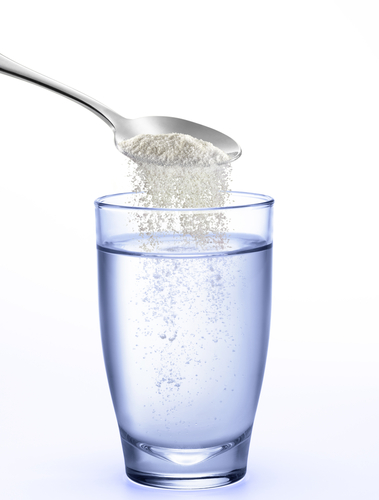Other than these, there are several other examples as well which are very common for household purposes:

• Saltwater is formed when we mix salt in water.
• Vinegar is obtained when we mix acetic acid in water.
• Tincture of iodine is formed when we dissolve crystals of iodine in alcohol.
• Mouthwash consists of a number of chemicals dissolved in water.

Important Topics of Chapter Solution

• Types of solutions
• Solubility
• Vapour pressure of liquid solutions
• Raoult's law
• Ideal and non-ideal solutions
• Colligative properties
• Abnormal molar mass
Prepare Online for JEE Main/NEET

Crack JEE 2021 with JEE/NEET Online Preparation Program

Overview of Chapter

There are various kinds of binary solutions exist in nature as given in the table below:

 Types of Solutions Solvent Solute Examples Gaseous Gas Gas Mixture of Oxygen and Nitrogen Gas Liquid Humidity Gas Solid Camphor in Nitrogen gas Liquid Liquid Gas Carbon dioxide in Water Liquid Liquid Milk dissolved in water Liquid Solid Sugar dissolved in water Solid Solid Gas Hydrogen in Palladium Solid Liquid An amalgam of mercury with sodium Solid Solid Brass (an alloy of copper and zinc)

In this chapter, there are various important topics that you must understand completely:

(i) Solubility: The maximum ability of a substance to get completely dissolved in a solvent. When the solute is solid and the solvent is liquid, then the only temperature affects the solubility. With the increase in temperature, the solubility increases. But pressure has no effect in this case as both solids and liquids are incompressible. But is the solute is gas, then pressure also is an important factor that plays major role insolubility. For this case, Henry's law is given which states that - "At constant temperature, the solubility of a gas is directly proportional to the partial pressure of a gas present above the solution".

(ii) Vapour pressure of liquid solutions: In this concept, you learn that the vapour pressure of volatile liquids in solution when taken in a closed vessel. This phenomenon is explained by Raoult's law, which states that - "The vapour pressure of each volatile liquid present in the solution is directly proportional to the mole fraction of that liquid present in solution".Mathematically, Raoult's law can be expressed as follows:

where, vapour pressure of pure component 1
= vapour pressure of pure component 2
= mole fraction of component 2

(iii) Ideal and non-ideal solutions: Ideal solutions are those solutions which obey Raoult's law at all ranges of concentrations. Whereas the non-ideal solutions are those which do not obey Raoult's law at all range of concentrations. The vapour pressure of non-ideal solutions are always higher or lower than as predicted by Raoult's law and thus we say that the solution is exhibiting the positive or negative deviation, respectively.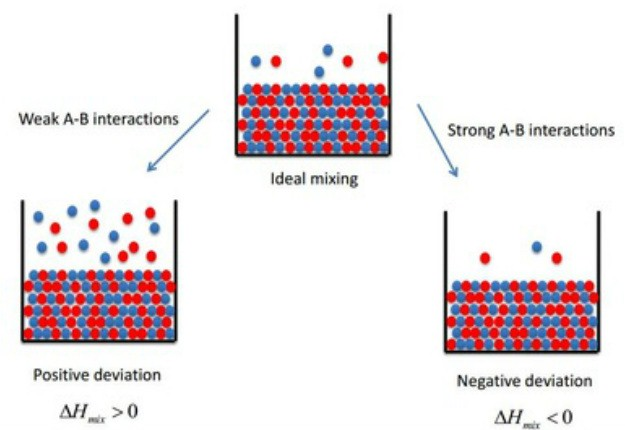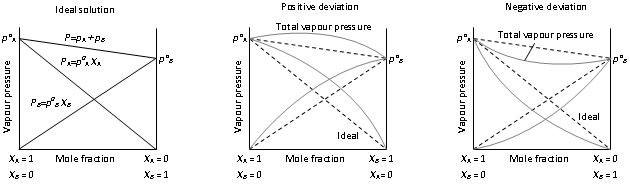(iv) Colligative properties: The properties of solutions which depend only on the number of solute particles present in the solution are known as colligative properties.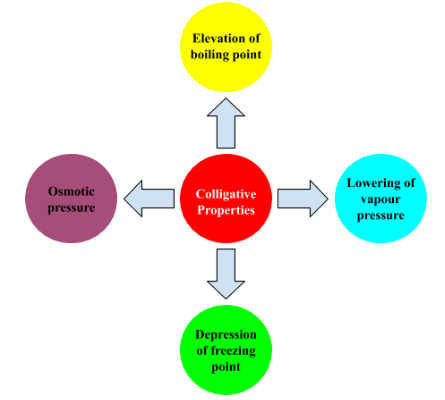• Relative lowering of vapour pressure: The actual vapour pressure of a solvent in a solution is less than that of the pure solvent. Raoult explained this behaviour and established that this lowering of vapour pressure depends only on the concentration of the solute particles. Mathematically, it can be expressed as follows:
• Elevation of the boiling point: The boiling point is the point of temperature when the vapour pressure of a substance is equal to the atmospheric pressure. This also depends upon the number of solute particles present in the solution.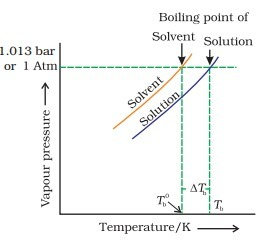Mathematically, it can be expressed as follows:

K= Boiling point elevation constant
• Depression of freezing point:

Abnormal Mass: This concept says, that when a solute is dissolved in a liquid then the solute does not dissociate completely as expected instead it dimerizes and thus the molar mass of solute become double. Such deviation of molar mass from the actual value is known as abnormal molar mass.

To deal with the case of an abnormal mass, a Vant Hoff factor was introduced in 1880, which mathematically is described as follows:

How to prepare for Solutions

• This chapter is a part of the physical chemistry and it is very important from the numerical point of view. The theory part is less but you simply need to work with the formulas and questions of this chapter.
• There are some common terms are used in this chapter like vapour pressure and mole fraction, so before coming to this chapter, first, you must finish the two basic chapters - "Mole concept" and "Gaseous state".
• You must have completely memorized all the formulas and must have the very good hold in solving the numericals.
• Usually, this chapter is liked by most of the students as it is very easy and straightforward. This chapter holds a good weight ok marks in Boards exams as well as in competitive exams like JEE and NEET.

Chemistry Chapter- wise Notes for Engineering and Medical Exams

 Chapters No. Chapters Name Chapter 1 Some basic concepts in chemistry Chapter 2 States of matter Chapter 3 Atomic Structure Chapter 5 Chemical Thermodynamics Chapter 6 Equilibrium Chapter 7 Redox Reaction and Electrochemistry Chapter 8 Chemical kinetics Chapter 9 Surface Chemistry Chapter 10 General Principle and processes of Isolation of metals Chapter 11 Classification of Elements and Periodic table Chapter 12 Hydrogen Chapter 13 p- block Elements Chapter 14 s-block Elements (Alkali and Alkaline Earth Metals) Chapter 15 Chemical Bonding and Molecular Structure Chapter 16 d- and f- BLOCK ELEMENTS Chapter 17 Coordination Compounds Chapter  18 Environmental Chemistry Chapter 19 Purification and Characterisation of Organic Compounds Chapter 20 Some Basic Principles of Organic Chemistry Chapter 21 Hydrocarbons Chapter 22 Organic Compounds containing Halogens Chapter 23 Organic Compounds containing Oxygen Chapter 24 Organic Compounds Containing Nitrogen Chapter 25 Polymers Chapter 26 Biomolecules Chapter 27 Chemistry in Everyday Life Chapter 28 Principles Related to Practical Chemistry

Topics from Solutions

• Different methods for expressing concentration of solution - molality, molarity, mole fraction, percentage (by volume and mass both) ( JEE Main, AP EAMCET Pharmacy, TS EAMCET Pharmacy ) (8 concepts)
• vapour pressure of solutions and Raoults Law ( JEE Main, AP EAMCET Pharmacy, TS EAMCET Pharmacy ) (6 concepts)
• Ideal and non-ideal solution ( JEE Main, AP EAMCET Pharmacy, TS EAMCET Pharmacy ) (9 concepts)
• plots for ideal and non-ideal solutions ( JEE Main, AP EAMCET Pharmacy, TS EAMCET Pharmacy ) (7 concepts)
• elevation of boiling point and osmotic pressure ( JEE Main, AP EAMCET Pharmacy, TS EAMCET Pharmacy ) (12 concepts)
• vapour pressure - composition ( JEE Main, AP EAMCET Pharmacy, TS EAMCET Pharmacy ) (6 concepts)
• Determination of molecular mass using colligative properties ( JEE Main, AP EAMCET Pharmacy, TS EAMCET Pharmacy ) (2 concepts)
• relative lowering of vapour pressure ( JEE Main, AP EAMCET Pharmacy, TS EAMCET Pharmacy ) (3 concepts)
• depression of freezing point ( JEE Main, AP EAMCET Pharmacy, TS EAMCET Pharmacy ) (7 concepts)
• vant Hoff factor and its significance. ( JEE Main, AP EAMCET Pharmacy, TS EAMCET Pharmacy ) (4 concepts)
• SOLUTIONS ( JEE Main, AP EAMCET Pharmacy, TS EAMCET Pharmacy ) (2 concepts)
• VAPOUR PRESSURE OF LIQUID SOLUTIONS ( JEE Main, AP EAMCET Pharmacy, TS EAMCET Pharmacy ) (4 concepts)
• IDEAL AND NON IDEAL SOLUTIONS ( JEE Main, AP EAMCET Pharmacy, TS EAMCET Pharmacy ) (5 concepts)
• COLLIGATIVE PROPERTIES ( JEE Main, AP EAMCET Pharmacy, TS EAMCET Pharmacy ) (6 concepts)
• VAN'T HOFF FACTOR ( JEE Main, AP EAMCET Pharmacy, TS EAMCET Pharmacy ) (5 concepts)

Important Books for Solutions

•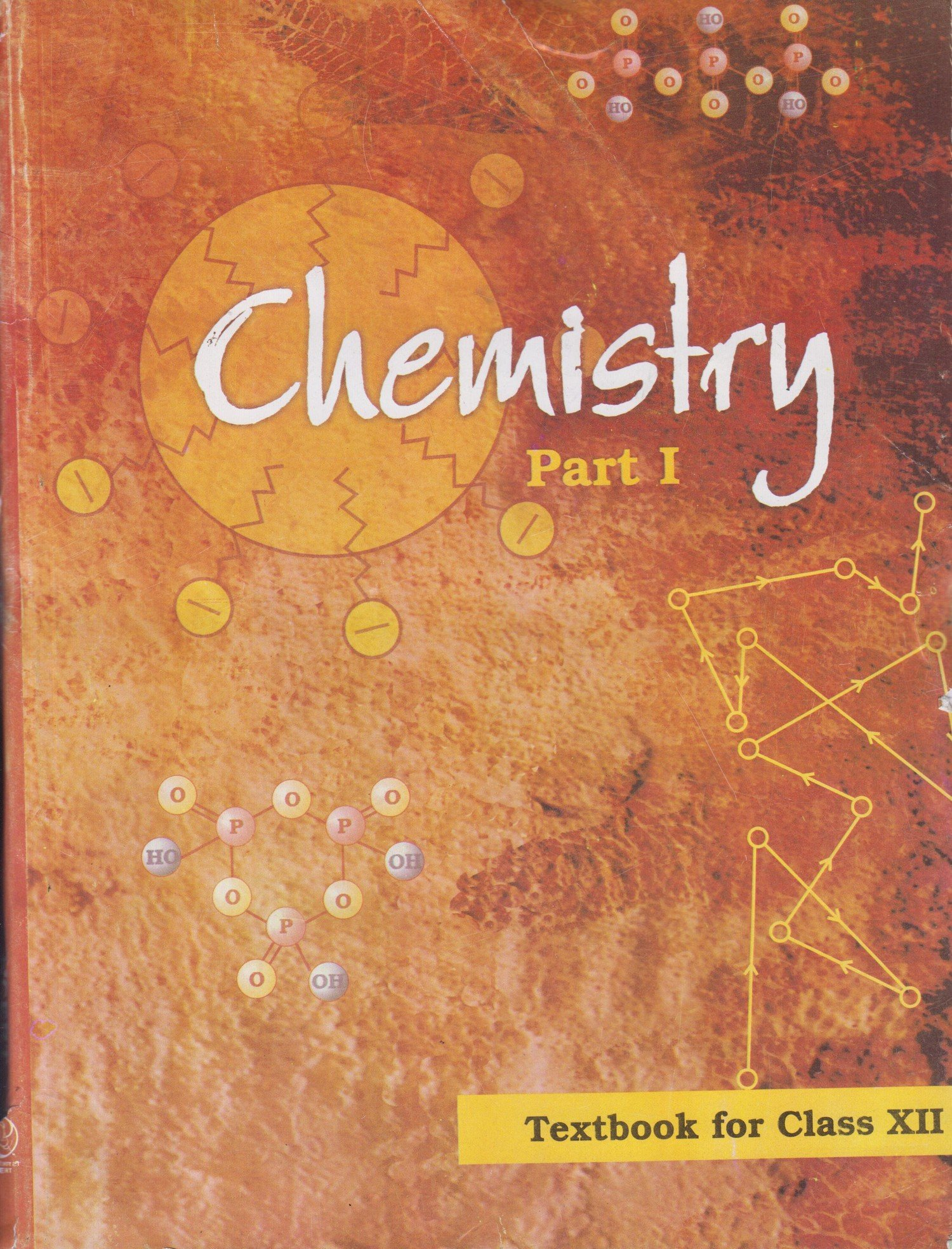•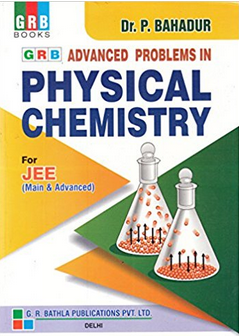Exams
Articles
Questions